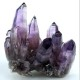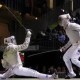# Can You Do This Math Exercise – Prime Factorization?

﻿

1. What is the prime factorization of 34 ?

A. 2 × 2 × 2 × 7

B. 2 × 17

C. 2 × 2 × 3 × 7 × 11

D. 2

E. 2 × 2 × 3 × 3

2. What is the prime factorization of 75 ?

A. 3 × 5 × 5

B. 2 × 2 × 3 × 7

C. 2 × 2 × 2 × 3 × 3

D. 2 × 2

E. 2 × 2 × 3

3. What is the prime factorization of 87 ?

A. 2 × 2 × 7

B. 2 × 2 × 2 × 3

C. 3 × 29

D. 2 × 5 × 11

E. 2 × 2

4. What is the prime factorization of 42 ?

A. 2 × 2 × 3

B. 2 × 3 × 7

C. 2 × 21

D. 2 × 2 × 2 × 3 × 3

E. 2 × 3

5. What is the prime factorization of 17 ?

A. 2 × 3 × 3

B. 2 × 2

C. 17

D. 2 × 2 × 2

E. 2 × 2 × 5 × 11

6. What is the prime factorization of 72 ?

A. 2 × 2 × 2

B. 2 × 2 × 3

C. 2 × 2 × 2 × 3 × 3

D. 2 × 2 × 18

E. 2

7. What is the prime factorization of 35 ?

A. 2 × 3 × 3

B. 2 × 2 × 2

C. 5 × 11

D. 5 × 7

E. 2 × 3 × 7

8. What is the prime factorization of 66 ?

A. 2 × 3 × 7

B. 2 × 2 × 2

C. 2 × 33

D. 2 × 3 × 11

E. 2 × 2

9. What is the prime factorization of 18 ?

A. 2 × 2 × 5 × 11 × 13

B. 2 × 2 × 3 × 3

C. 5 × 7

D. 2 × 2 × 2 × 3

E. 2 × 3 × 3

10. What is the prime factorization of 92 ?

A. 5 × 7

B. 2 × 46

C. 2 × 2 × 23

D. 2 × 2 × 2 × 5 × 11

E. 2

### 7 responses to “Can You Do This Math Exercise – Prime Factorization?”

1.malachite57

1. B
2. A
3. C
4. B
5. C
6. C
7. D
8. D
9. E
10. C
I feel very intelligent. 😀 (Not really!)

2.Emerald

What is this math prime factorization anyways?

3.Quartz

1.B 2.A 3.C 4.B 5.C 6.C 7.D 8.D 9.E 10.C Same as Diamond.

4.Diamond

4.B 5.C 6.C 7. C 8.D 9.E 10.C

•Diamond

and 7 should be D!!!!

5.Diamond

1. A 2.A 3.C

•Diamond

Oops! TYPO!! 1.B not 1.A